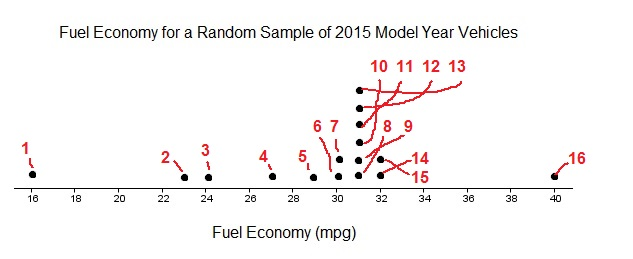# How to read a dotplot

As you will see below, dotplots are some of the easiest to read plots in statistics. That is, they are easy to read if you keep one thing in mind: each data value gets a dot and dots are stacked*. Of course, if you just came from our article on how to make dotplots, then you already know that. To understand how to read a dotplot, we will look at an example data set and see what kinds of questions we can answer.

## Answering questions from a dotplot

The dotplot below represents the fuel economy (in miles per gallon) for a sample of 2015 model year cars.Use this dotplot to answer the following questions.

(a) How many vehicles are represented in the sample?
(b) What was the smallest value in the data set?
(c) How many vehicles in the sample get 31 mpg?
(d) What is the median mpg for vehicles in this sample?

This looks like a lot – let’s try each one individually.

### (a) How many vehicles are represented in the sample?Remembering our rule (each dot is a single data value), we can just count the number of dots. If you do that, you will find that there are 16. Therefore, we have 16 vehicles represented in the sample.

### (b) What was the smallest value in the data set?

The horizontal scale represents the fuel economy, which are the values that make up our data set. The dot that represents the smallest value is just above the 16. So, 16 mpg is the smallest value in our data set. Phew – wouldn’t want to be filling up the tank for that car!
(if you are curious, that is the fuel economy for an Aston Martin V8 Vantage S – perhaps if that is the car you are driving, you aren’t too worried about fuel economy after all?)

### (c) How many vehicles in the sample get 31 mpg?The scale used here counts by 2; starting at 16. So, dots representing 31 are in between dots representing 30 and 32 on the scale. Counting these, there are 6 total dots. So, 6 of the sampled vehicles get 31 mpg.

### (d) What is the median mpg for vehicles in this sample?

The median represent the middle value of a data set when it is placed in order from smallest to largest. If there are an even number of data values, like we have here (remember there are a total of 16 vehicles represented), then it is the average of the two middle values. For a data set with 16 values, this would be the average of the 8th and 9th value.

If you count from left to right (since the values have to be in order to find the median), the 8th and 9th values are both 31.So, the median is $$\dfrac{31+31}{2}=31$$ mpg.

You could also do this by listing out each of the values and then finding the average of the middle two. However, the more values there are, the more difficult this will be.